Math worksheetsCustom math worksheets at your fingertips

# Details for problem "Dividing fractions fill in the blanks"

Quickname: 5949

## Summary

In a division term of two fractions, blanks have to be filled in.

## Example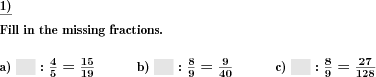## Description

A quotient term with two fractions is given, as is the result. One of the fractions is replaced by a blank space. The correct fraction has to be filled in.

The number range for the numerators and denominators of the reduced fractions can be specified. After converting the result to like quantities, the numerator and denominators of the unreduced result may be up to the square of the range.

Improper fractions can be allowed, disallowed or enforced. In case they may occur, it can be chosen whether they will be output as improper fractions or mixed numbers.

The number of problems is selectable.

Download free worksheets for this math problem here. There is a second sheet with the solutions. Just click on the respective link.Worksheet 1Solution sheetWorksheet 2Solution sheetWorksheet 3Solution sheet

If you can not see the solution sheets for download, they may be filtered out by an ad blocker that you may have installed. If this is the case, please allow ads for this page and reload the page. The solution sheets will then reappear.

• Do the sample worksheets do not really fit?
• Do you need more math worksheets, with a different level of difficulty?
• Would you like to combine different problems on a worksheet and adjust them to your needs?
• As a teacher, you can put together your own worksheets using the automatically generated math problems provided.
With a free initial credit, you can start creating your own math worksheets in a few minutes.

It does not cost anything to try! Register here, to create custom worksheets now!

## Customization options for this problem

ParameterPossible values
Number of problems1, 2, 3, 4, 5, 6, 7, 8, 9, 10
Number range10, 20, 30, 40, 50, 100, 200, 500, 1000, 10000, 100000
Improper fractionsNo, Yes, as a fraction, Yes, as a mixed number

## Similar problems

as a plain variant, division of fractionsDivision of two fractions.Dividing fractions

## Other types of problems that appear on worksheets with this problem:

RelevanceNameDescriptionQuicknameExample
****Add Sub times tableAddition and subtraction of times table products7300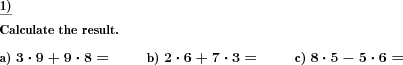****Add Sub times table same factorAddition and subtraction of times table products for a single number one to ten5139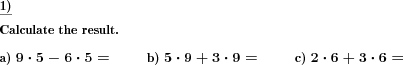****Dividing fractionsDivision of two fractions.5923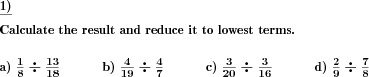****Dividing fractions by whole numbersA fraction has to be divided by a whole number.5220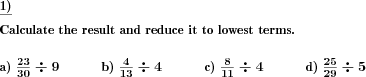Deutsche Version dieser Aufgabe
These informational pages with samples describe math problems that can be combined on custom math worksheets with solutions for home and school use.